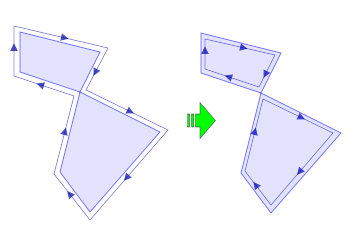# Clipper.StrictlySimple

Del.» property StrictlySimple: boolean; override;

C++ » void StrictlySimple(bool value);

C#  » public bool StrictlySimple { get {} set {} };

Terminology:

• A simple polygon is one that does not self-intersect.
• A weakly simple polygon is a simple polygon that contains 'touching' vertices, or 'touching' edges.
• A strictly simple polygon is a simple polygon that does not contain 'touching' vertices, or 'touching' edges.

Vertices 'touch' if they share the same coordinates (and are not adjacent). An edge touches another if one of its end vertices touches another edge excluding its adjacent edges, or if they are co-linear and overlapping (including adjacent edges).

Polygons returned by clipping operations (see Clipper.Execute()) should always be simple polygons. When the StrictlySimply property is enabled, polygons returned will be strictly simple, otherwise they may be weakly simple. It's computationally expensive ensuring polygons are strictly simple and so this property is disabled by default.

Note: There's currently no guarantee that polygons will be strictly simple since 'simplifying' is still a work in progress.In the image above, the two examples show weakly simple polygons being broken into two strictly simple polygons. (The outlines with arrows are intended to aid visualizing vertex order.)

See also the article on Simple Polygons on Wikipedia.